# We served 2,800 customers last month and 3,200 customers this month. What percentage increase is that?

This calculation requires three steps.

First calculate the difference between the two numbers. Then, calculate this value as a 'fraction' of the earlier number. Finally ask Excel to display this result as a percentage.

The difference between the two numbers is always the later or more recent number minus the earlier or older number.

In the example below, customer numbers have increased from 2,800 last month to 3,200 this month. First, calculate the difference.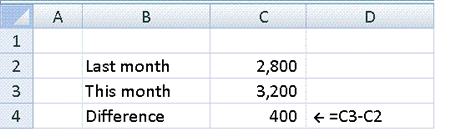Next, take the difference between the two numbers and divide by the original number.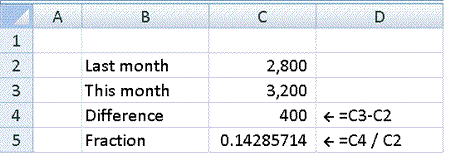Finally, change the format of the answer so that Excel displays it as a percentage. This can be done using the percentage icon on the toolbar.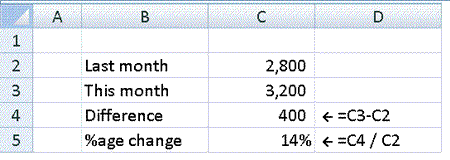It is usually worthwhile increasing the number of decimal places displayed so that a more accurate value is shown.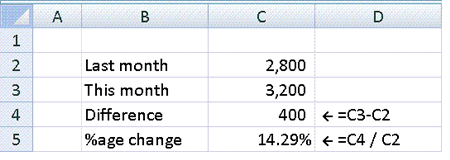Customer numbers changing from 2,800 to 3,200 represents an increase of 14.29%.

#### We served 3,500 customers last month and 3,400 customers this month. What percentage decrease is that?

Where the number decreases, the steps are the same.

In the example below, customer numbers have decreased from 3,500 last month to 3,400 this month. First, calculate the difference.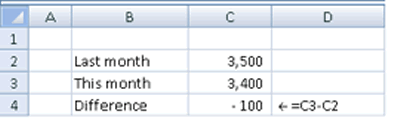Here, the difference is a negative number, representing a decrease in numbers.

Next, take the difference between the two numbers and divide by the original number.Finally, change the format of the answer so that Excel displays it as a percentage. This can be done using the percentage icon on the toolbar.Again, it is usually worthwhile increasing the number of decimal places displayed so that a more accurate value is shown.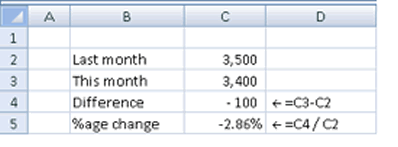Customer numbers changing from 3,500 to 3,400 represents a decrease of 2.86%.

Try it yourself using the examples below...